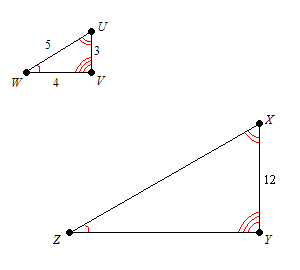# Scale Factor

Suppose you have two similar figures , one larger than the other. The scale factor is the ratio of the length of a side of one figure to the length of the corresponding side of the other figure.

Example:Here, $\frac{XY}{UV}=\frac{12}{3}=4$ . So, the scale factor is $4$ .

Note that when a two-dimensional figure is enlarged ( dilated ) by a scale factor of $k$ , the area of the figure is changed by a factor of ${k}^{2}$ . In case of three-dimensional figures, when a figure is dilated by a scale factor of $k$ , the volume is changed by a factor of ${k}^{3}$ .